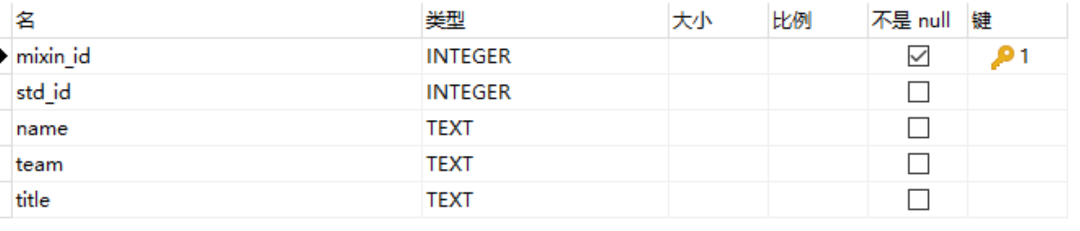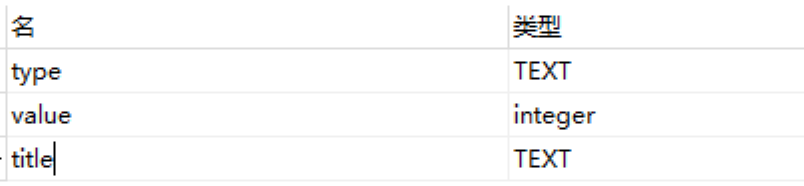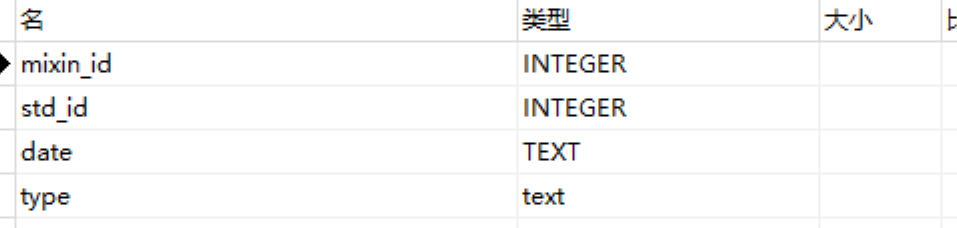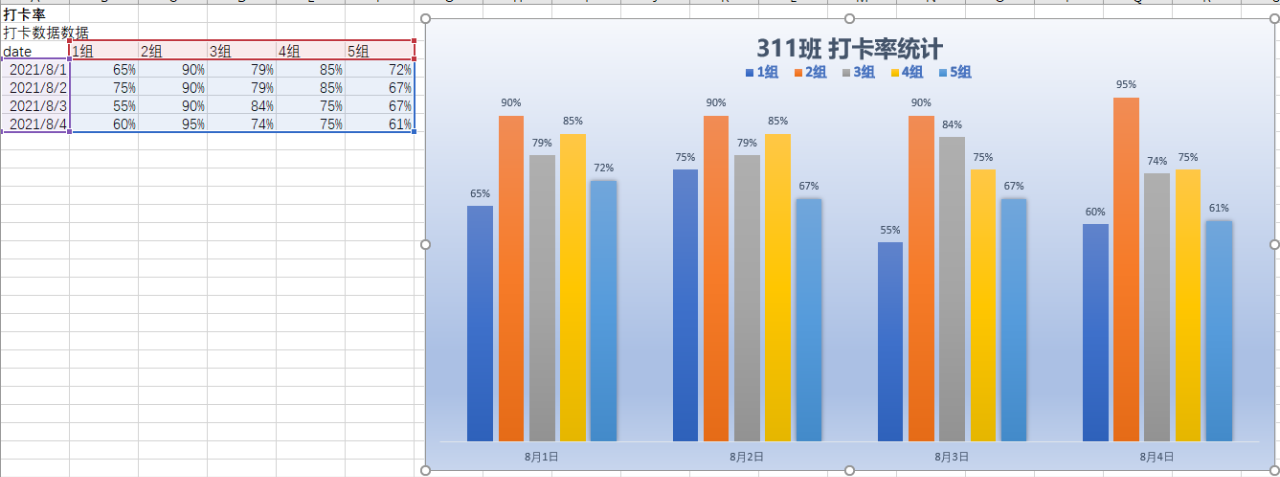2022
07-18

# YYDS! Python 帮我扛起运营大旗！## 基础整理

SQLite 很轻，不需要服务器，但功能与 MySQL类似。

`pip install sqlite3`

```import sqlite3
conn = sqlite3.connect('database.db')```

```# 创建一个游标 cur = con.cursor() # 执行SQl 语句创建库表 cur.execute('''CREATE TABLE stocks
(date text, trans text, symbol text, qty real, price real)''') # 向库表中插入数据 cur.execute("INSERT INTO stocks VALUES ('2006-01-05','BUY','RHAT',100,35.14)") # 提交更改结果 con.commit() # 关闭链接 con.close()```

```class DBSqlite:     def __init__(self, db):         super().__init__()
self.db = db
self._conn = None     def __del__(self):         if self._conn:
self._conn.close()

def _get_conn(self):         if not self._conn:
self._conn = sqlite3.connect(self.db)
self._conn.row_factory = sqlite3.Row
return self._conn

def _query(self, sql):         conn = self._get_conn()
cur = conn.cursor()
rows = []
for row in cur.execute(sql):
rows.append(row)
cur.close()
return rows

def de(self, sql):         conn = self._get_conn()
cur = conn.cursor()
for s in sql.split(";"):
cur.execute(s)
conn.commit()
cur.close()
return True     def insert(self, table, rows):         conn = self._get_conn()
cur = conn.cursor()
cur.executemany("insert into %s values (%s)" % (table, ("?,"*len(rows))[:-1]), rows)
conn.commit()
cur.close()
return True     def query(self, sql):         conn = self._get_conn()
cur = conn.cursor()
cur.execute(sql)
rows = cur.fetchall()
cur.close()
return rows```
• 封装了基本操作，de 为执行一个数据库操作，insert 为插入数据，query 执行一个查询
• 需要注意的是 _get_conn 中的 self._conn.row_factory = sqlite3.Row 语句，作用时执行查询后，返回的结果会被转化为 sqlite.Row 对象，以便通过字段名来读取值，详细参加 row_factory。

## 结构设计• mixin_id 是用户注册App的id
• std_id 为打卡系统的id
• team 为小组名
• title 为职务活动表
• type 为活动类型
• value 为活动积分
• tilte 为该活动对于的职务• mixin_id 为用户id，std_id 其实是没必要的，不过录入打卡记录时顺带记录了
• date 为活动发生的日期
• type 为活动内容

## 数据收集

1. 因为Excel 处理需要安装额外库，也没有文本文件处理方便。
2. 另外未来考虑做成 Web 系统，所以没有做 Excel 的进一步扩展。

```def record_check(rows):     dbrows = []
for row in rows:
u = get_user(std_id=int(row))
if u:
if row != "×":
dbrows.append((u['mixin_id'], u['std_id'], row, "打卡", 1, row, None))
else:
print("没有找到用户：", row)
if len(dbrows) > 0:
db.insert("tprj_activity", dbrows)
return dbrows```
• record_check 方法是用来记录打开记录的，参数 rows 是从打开后台抓取的数据
• get_user 是可以根据打卡用户的 id，从用户表中找到用户记录，然后结合打卡记录，补全打卡记录
• db 是 上面提到的 DBSqlite 的一个实例，调用其 insert 方法将数据插入数据库

```def merge_activity(datafilename):     rows = []
with open(datafilename, 'r', encoding='utf-8') as check_f:
data = {}
for line in check_f:
linedata = line[:-1].split('\t')
date = linedata.replace("/","-")
userinfo = linedata.split("/")
team = userinfo
name, mixin_id, std_id = userinfo.split('-')
atype = linedata
rows.append((mixin_id, date, atype))
...```

## 自动核算

```INSERT INTO tprj_user_score_detail  SELECT a.mixin_id, sum(s.value), u.team, '成员', a.date  FROM     tprj_activity a
LEFT JOIN tprj_user u ON a.mixin_id = u.mixin_id
LEFT JOIN tbas_score s ON a.type = s.type  WHERE s.title = '成员'  GROUP BY     a.mixin_id,
u.team,
u.title,
a.date ```
• 查询所有职务属于 成员 的活动积分，插入成员积分明细表
• tprj_activity 为活动记录表，与 tprj_user 用户表链接，然后再链接上活动表 tbas_score，作用是对活动类做约束
• where 条件中，限制活动类型必须为 成员 活动
• sum(s.value) 为一个成员的当日积分合计，日期 体现在 group by 的条件中了

```import sql
...
db.de(sql.user_score_detail)
...```

```def cal_check_rate():     ## 计算打卡率     team_member = {}
for r in db.query(sql.team_member_count):
team_member[r['team']] = r['mcount']
dbrows = []
for r in db.query(sql.team_check_count):
dbrows.append((r['team'], r['date'], round((r['checkcount']/team_member[r['team']])*100)))
if len(dbrows) > 0:
db.insert("tprj_team_check_rate", dbrows)
return dbrows```
• team_member_count 语句语句获取各组的人数，因为可能有人没有注册打卡。只通过打卡记录获取组内人数，不严谨。
• team_check_count 语句是按组和日期分类核算出的组打卡数
• 打卡率公式为：（打卡个数/组内人数） * 100%
• 将计算好的打卡率，按日期存入 dbrows，最后插入数据库

## 报表导出

```SELECT
date,
max(case when team ='1组' then rate else 0 end) as '1组',
max(case when team ='2组' then rate else 0 end) as '2组',
max(case when team ='3组' then rate else 0 end) as '3组',
max(case when team ='4组' then rate else 0 end) as '4组',
max(case when team ='5组' then rate else 0 end) as '5组' FROM tprj_team_check_rate GROUP BY date ```
• tprj_team_check_rate 是用于按组和日期存放打卡率
• select 语句中，使用了行转列的技巧，使得结果为 第一列为日期，后面列为各个组，这样是为了绘制成图表方便

1. 操作 Excel 比较费劲，调试工作量大
2. 我有更大的打算，即最终实现为在线版的，所以花费大量时间不值得

```def show_check_rate():     data = db.qj(sql.check_rate_show)
result = []
# 处理表头     line = '\t'.join(data.keys()) + "\n"     result.append(line)

# 生成表头     for d in data:
row = []
for k in d.keys():
if k != 'date':
row.append(str(d[k]) + "%")
else:
row.append(d[k])
line = '\t'.join(row) + "\n"         result.append(line)
result.append('\n')
return result```
• check_rate_show 执行 Sql 获得数据
• 从数据中获取表头信息，做成一行记录，请注意字段的分隔为 tab 符，这样是为了方便直接粘贴到 Excel 中
• 取出数据中的每一行，做成表体数据行
• 最后再加入一个回车，这是为了和其他的输出分隔开

```filename = "result_%s.txt" % today.strftime("%Y-%m-%d %H_%M_%S") with open(filename, 'w', encoding='utf-8') as r:
r.writelines(show_check_rate()) # 打卡率     r.writelines(show_member_score()) # 成员积分     ...```
• filename 为要写入的文本文件，这里利用当前时间作为文件名，是为了不重复
• 打开文件，用 writelines 方法将返回的行写入文件中
• 这里还可以调用其他产生输出方法，将结果写入文件

```date	1组	2组	3组	4组	5组
2021-08-01	65%	90%	79%	85%	72%
2021-08-02	75%	90%	79%	85%	67%
2021-08-03	55%	90%	84%	75%	67%
2021-08-04	60%	95%	74%	75%	61%```## 日常维护

```score_type_temp = "max(case when type ='{atype}' then num else 0 end) as '{atype}'" types = db.query("select type, value from tbas_score where title='%s'" % title)
temps = [] for t in types:
temps.append(sql.score_type_temp.format(atype=t['type']))

allsql = sql.member_score.format(",\n".join(temps))```

## 总结